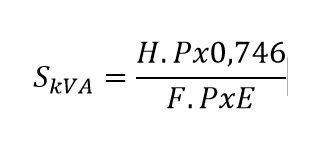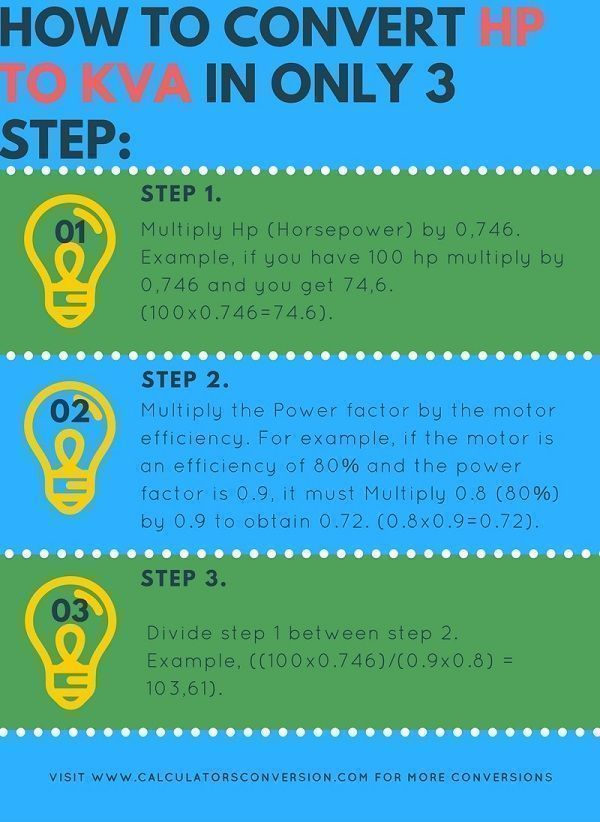# HP to kVA – Online Convert free

Calculator from Hp to kVA can be somewhat overwhelming, however with this tool you could convert with just 1 click.

You will have the step-by-step explanation of how the conversion is done and what is the formula used for the calculation.

With the idea of ​​making things even easier, we present some illustrative examples, equivalence tables from Hp to kVA and common values ​​for the efficiency and power factor of electric motors.

## ⚡HP formula to kVA

The HP to kVA formula is applied to electric motors that can be single-phase, two-phase or three-phase, the difference of this formula with that of HP at kW is that it includes the  power factor .

##Where:

• S (kVA) = Kilovolt-Amps. It is the apparent power we wish to find.
• HP (Horsepower) = Horsepower, data that you can see on the nameplate of the electric motors or by doing a search of the engine reference on the internet.
• E = Efficiency of the electric motor. Efficiency is the ratio between the mechanical power delivered by the motor (output) and the electrical power supplied to the motor (input). Efficiency = (Mechanical power output / Electric power input) x 100% Therefore, a motor that It is efficient at 85% converting 85% percent of the electrical energy input into mechanical energy, the remaining 15% of the electrical energy dissipates as heat, evidenced by an increase in the engine temperature. Some reference values ​​for  motor efficiency  can be found in countless internet tables.
• FP = Power factor. The  power factor  is an expression of energy efficiency. It is usually expressed as an integer from 0 to 1, and the lower the value, the less efficient is the use of energy. It is a value of each team and can be obtained from the characteristics of the same or from the catalogs.

## ⭐How to convert Hp to kVA in only 3 step### Step 1 (Multiply Hp x 0.746)

Multiply Hp (Horsepower) by 0.746. Example, if you have 100Hp (horsepower) multiply by 0.746 and get 74.6. (100 × 0.746 = 74.6).

### Step 2 (Efficiency x FP)

Multiply the power factor by the efficiency of the engine. For example, if the engine has an efficiency of 80% and the power factor is 0.9, you must Multiply 0.8 (80%) by 0.9 to get 0.72. (0.8 × 0.9 = 0.72).

### Step 3 (Divide step 1 and 2)

Divide step 1 by step 2. Example, ((100 × 0.746) / (0.9 × 0.8) = 103.61).

## ✍Examples of conversions from Hp to kVA

### Example No. 1 (Rock Crusher)

A rock crusher has a power of 40Hp, a power factor of 0.86 and an efficiency of 89%, how many kVA does the rock crusher have?

Rta: // The first step is to multiply 40 × 0.746, which will result in 29.84, then this result should be divided between the multiplication of the Power Factor and efficiency, as follows: 40 × 0.746 / (0.86 × 89 %) = 38.99kVA

### Example No. 2 (Steel bender)

A steel bending machine has a power of 20Hp, with a power factor of 0.82 and an efficiency of 84%, how many kVA will the steel bender have?

Rta: // Hp must be multiplied by 0.746, resulting in 14.92, then the power factor must be multiplied by efficiency (this value must be in decimals and not as a percentage, to pass it simply divide 84% by 100, which gives as a result 0.84), continuing with the operation the result will be: 0.688, then you must divide the first result by the second, as follows: 14.92 / 0.68 = 21.15kVA.

### Example No. 3 (Electric Curtain)

An engine for an electric curtain has a power of 7.4Hp, a power factor of 0.88 and an efficiency of 90%, how many kVA does the curtain motor have?

Rta: // It’s simple, you just have to enter the previous parameters to the calculator shown in this article and you give it to calculate, the result will be 6.97kVA.

## ☝Difference and explanation of Hp and kVA

Horsepower is a unit of mechanical power, while kVA (kilovolt-amps) is a unit used to classify electrical power.

In electrical terms, 1 horsepower equals 746 watts, but the conversion of Hp to kVA also requires knowing the power factor of the electrical device and efficiency.

An industry standard average rating of 0.8 power factor (for motors) or 0.9 for other electrical devices can be used if the actual power factor is unknown, as long as efficiency can be used 80 %. The use of the power factor and efficiency in conjunction with the known Hp will give a rough but reliable understanding of the power equivalence in kVA.

## 🔥 Most common conversions from Hp to kVA

### 1 Hp to how many kVA is it equivalent?

The answer is 1.17kVA, taking into account a power factor of 0.8 and an engine efficiency of 80%.

### 15hp to kVA

With a power factor of 0.85 and an efficiency of 88%, the answer is 14.96kVA.

### 100hp to kVA

100Hp is equal to 103kVA, with a power factor of 0.85 and an efficiency of 85%.

### 150 hp how many kVA are they?

They are 138kVA, with an efficiency of 90% and a power factor of 0.9.

### 5.5 HP to kVA

5.83kVA, with an efficiency of 88% and a power factor of 0.8

### 2.2 HP to kVA

2.28kVA (0.8 power factor) and 90% efficiency

## 🎯Table Hp to kVA of conversion, equivalence, transformation (Efficiency of 85%, power factor of 0.88)

 How many Hp are: Equivalence in kVA: 1 Hp Equivalent to 0.997 kVA 2 Hp 1,995 kVA 3 Hp 2,992 kVA 4 Hp 3,989 kVA 5 Hp 4,987 kVA 6 Hp 5,984 kVA 7 Hp 6,981 kVA 8 Hp 7,979 kVA 9 Hp 8,976 kVA 10 Hp 9,973 kVA 20 Hp 19,947 kVA 30 Hp 29,920 kVA 40 Hp 39,893 kVA 50 Hp 49,866 kVA 60 Hp 59,840 kVA 70 Hp 69,813 kVA 80 Hp 79,786 kVA 90 Hp 89,759 kVA 100 Hp 99,733 kVA 200 Hp 199,465 kVA 300 Hp 299.198 kVA 400 Hp 398,930 kVA 500 Hp 498,663 kVA 600 Hp 598.396 kVA 700 Hp 698,128 kVA 800 Hp 797,861 kVA 900 Hp 897,594 kVA 1000 Hp 997.326 kVA 1100 Hp 1097.059 kVA 1200 Hp 1196,791 kVA 1300 Hp 1296.524 kVA 1400 Hp 1396.257 kVA 1500 Hp 1495.989 kVA 1600 Hp 1595,722 kVA 1700 Hp 1695,455 kVA 1800 Hp 1795,187 kVA

Note : The previous conversions are made with a power factor of 0.88, and an efficiency of 85%. For different variables you should use the calculator that appears at the beginning.

## 👌How to use the calculator from Hp to kVA

The use of this calculator is very simple, the first thing you should do is enter the engine Hp, this value is found on the motor nameplate or in the equivalence tables, you must keep in mind that the power of the engines is defined in Hp for the United States and some other countries, while in Europe the power of the engines in kW is used.

Then you must enter the efficiency of the motor , this value is characteristic of the motor and is a measure of the effectiveness with which electrical energy is converted into mechanical energy.

Finally you must choose the power factor , this factor indicates the efficiency with which you use electricity.

After having everything ready you must click on “Calculate”

Spanish version Hp to kVA

Rate this calculator Hp to kVA:  [kkstarratings]